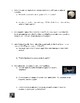# The Ultimate Orbital Mechanics WorksheetSubject
Resource Type
File Type

PDF

(38 MB|15 pages)
Standards
NGSSHS-PS2-4
NGSSHS-PS2-1
NGSSHS-ESS1-4
• Product Description
• StandardsNEW

This file contains 30 detailed Orbital Mechanics problems. Each problem uses real-world data and will have your students applying Kepler & Newton's laws. Orbital Mechanics is apart of every Physics & Astronomy curriculum and the ideas reinforced in the worksheet are covered on my Ultimate Rocket Science Guide PowerPoint. These problems have an answer key and a step by step solution guide is attached to the end as well. The idea of Orbital Mechanics is also a common topic on End of Course (EOC) and Advanced Placement (AP) Exams.

Topics

• Newton's Laws
• Newton's Universal Law of Gravity
• Kepler's Laws of Planetary Motion
• Orbital Velocity
• Escape Velocity
• Local Acceleration of Gravity
• Rocket Science!
NGSSHS-PS2-4
Use mathematical representations of Newton’s Law of Gravitation and Coulomb’s Law to describe and predict the gravitational and electrostatic forces between objects. Emphasis is on both quantitative and conceptual descriptions of gravitational and electric fields. Assessment is limited to systems with two objects.
NGSSHS-PS2-1
Analyze data to support the claim that Newton’s second law of motion describes the mathematical relationship among the net force on a macroscopic object, its mass, and its acceleration. Assessment is limited to one-dimensional motion and to macroscopic objects moving at non-relativistic speeds. Examples of data could include tables or graphs of position or velocity as a function of time for objects subject to a net unbalanced force, such as a falling object, an object sliding down a ramp, or a moving object being pulled by a constant force.
NGSSHS-ESS1-4
Use mathematical or computational representations to predict the motion of orbiting objects in the solar system. Emphasis is on Newtonian gravitational laws governing orbital motions, which apply to human-made satellites as well as planets and moons. Mathematical representations for the gravitational attraction of bodies and Kepler’s Laws of orbital motions should not deal with more than two bodies, nor involve calculus.
Total Pages
15 pages
Included
Teaching Duration
N/A
Report this Resource to TpT
Reported resources will be reviewed by our team. Report this resource to let us know if this resource violates TpT’s content guidelines.